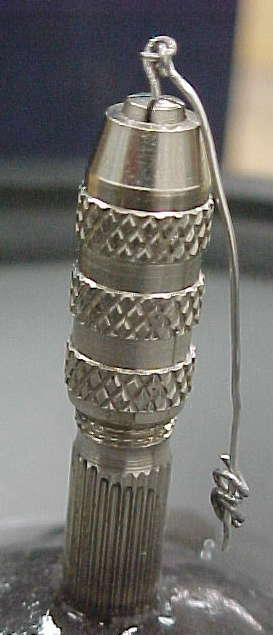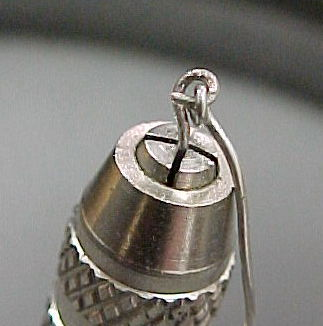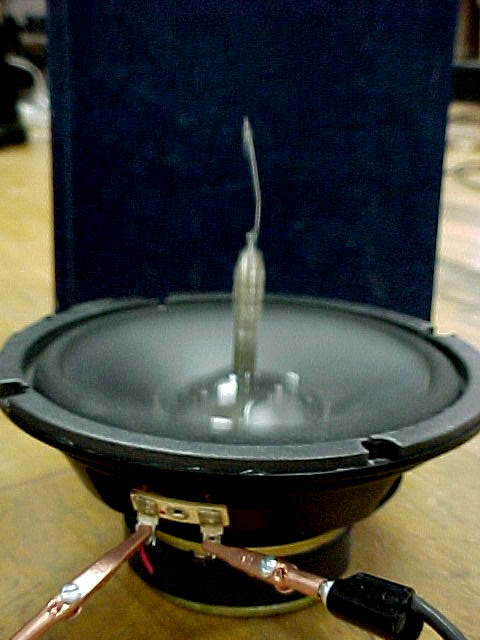# Experiment of The Month

## Dynamic Stabilization

In ordinary terms, a stable system in one which has a preferred configuration (such as a pendulum hanging straight down) to which it returns if it is is perturbed so that its configuration is slightly different from the preferred configuration. After the perturbation, the system typically oscillates about the preferred configuration and settles towards it as energy is dissipated.

The force which acts to return an ordinary pendulum to its preferred (equilibrium) configuration is a function of position only. This month's demonstration involves a return-force which depends on both position and time. It turns out that, for such a time-dependent force, a new preferred configuration becomes available. Because the time dependence is essential, this new preferred configuration is said to be a "dynamically stable" state, rather than an equilibrium state.

Dr. Miziumski recently built an inverted pendulum, which is a simple demonstration of dynamic stabilization, from spare parts lying around our advanced laboratory. The photos below show the device at rest. The pendulum is the wire with a bit of extra wire twisted at the lower end. In these photographs the pendulum is not inverted. It hangs (more or less) down from the little wire link.The wire link is gripped in a pin vise, and the pin vise in turn is glued to the cone of a loudspeaker. This part of the system was a spare from one of our Foucault pendulum drivers.When the loudspeaker is driven with a frequency of about 30 Hz, the pendulum will stabilize in an inverted configuration, as shown in the figure below:The pin vise is now blurred because it is vibrating up and down with an amplitude of a few millimeters. The little pendulum can be seen standing upright.

It turns out that the physics of this motion is the same as the physics for the driven Foucault pendulum. In both cases the acceleration of the pivot point can be understood to generate an oscillatory artificial gravity, in the frame of reference of the pivot.

For the Foucault pendulum, this artificial gravity adds energy to the oscillation about the ordinary equilibrium of the "regular" pendulum. For the inverted pendulum, we can understand the torques which drive the pendulum to stand up. The argument is made in the literature.

Here, we will present example of the forces which establish the dynamic "equilibrium" of the inverted pendulum, based on Dr. Mizumski's discussion in lab. Suppose that the pendulum mass is all at the end of the wire, a distance L from the pivot. The pendulum is stationary and the wire makes an angle of 30 degrees with the vertical. We consider a two-step iteration of the effect of torque on position.

Suppose that the artificial gravity in the accelerated frame is directed up. A torque is exerted which reduces the angle. The moment arm for the torque is Lsin30. Suppose that the pendulum is next at rest at an angle of 29 degrees when the artificial gravity reverses direction, becoming downward. Now there is a torque tending to increase the angle. However the torque is now smaller, because the moment arm is Lsin29 . The displacement toward larger angles is not as big. When this sequence is repeated, the angle tends to zero (provided that some dissipation is included).

A similar argument can be repeated if the initial artificial gravity points downward. Then the upward artificial gravity is applied to a moment arm of (say) Lsin31 and the upward torque is again the dominant torque.

Note. For a frequency of 30 Hz and amplitude of 3mm, the maximum acceleration is somewhat greater than 10 m/sec2.

## Experiment Of The Month

View past labs experiments.